# Sample Data: Solar System Planets and Moons

Sample dataset containing the mass and radius of planets and moons in the Solar System

Originator: Wolfram Research

(3 columns, 8 rows)

## Examples

### Basic Examples

Retrieve the ResourceObject:

 In:=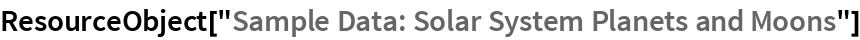Out=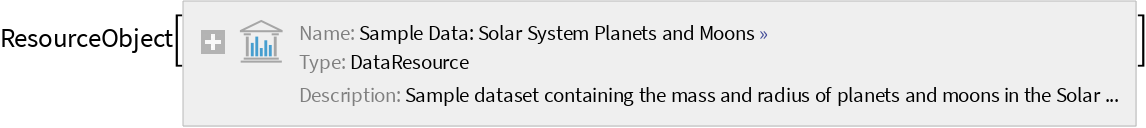View the data:

 In:=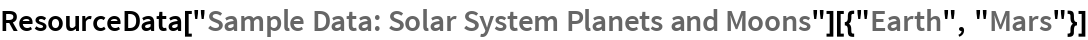Out=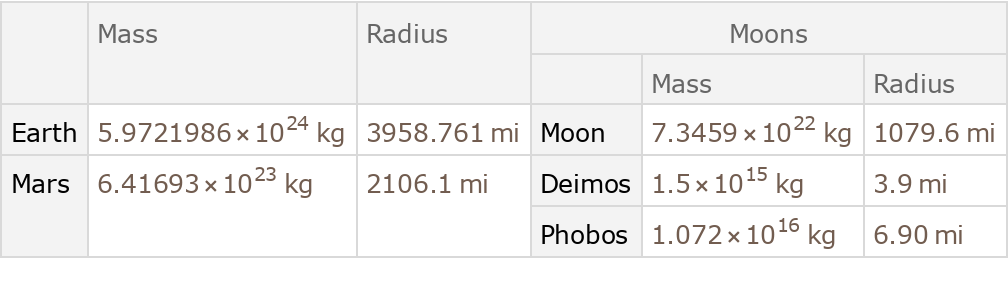### Analysis

Find the radii of all the planets:

 In:=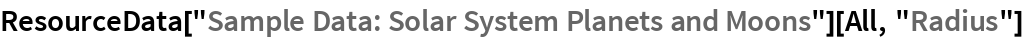Out=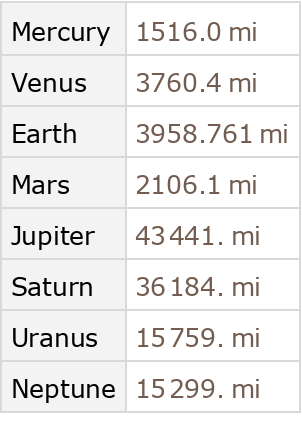Find the total mass of all moons for each planet:

 In:=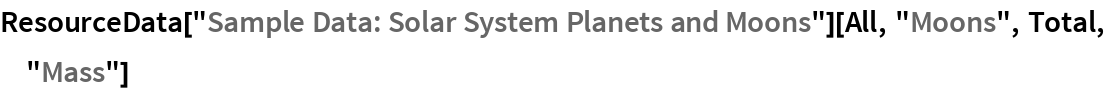Out=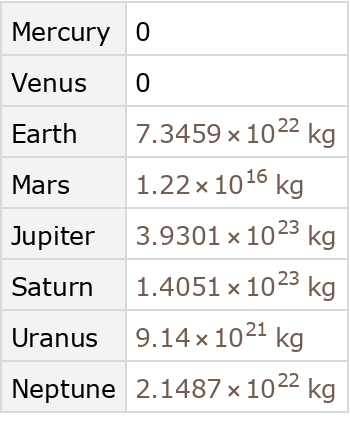Get a dataset with moons that are more than 1% of the mass of Earth:

 In:=Out=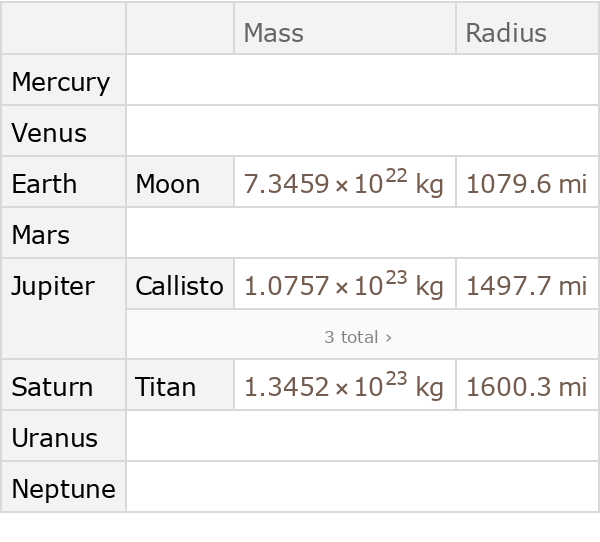Wolfram Research, "Sample Data: Solar System Planets and Moons" from the Wolfram Data Repository (2017)